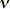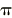Constants Topics: Values Energy Equivalents Searchable Bibliography Background Constants Bibliography Constants, Units & Uncertainty home pageIntroduction to the constants for nonexperts

The following material is intended to give nonexperts insight into the general subject of fundamental physical constants. It is adopted from an article written by Barry N. Taylor in about 1971 for the 15th edition of the Encyclopaedia Britannica © 1974 , and is reproduced with permission. Although a considerable portion of the article is now out-of-date, the introductory and historical portions given here remain generally valid. Note, however, that the terms accuracy and uncertainty are used in the text in a manner that is not always consistent with current practice. This also applies to other terms such as parts per million and its symbol ppm, and the now obsolete terms atomic weight and molecular weight. To add a more modern flavor to the material, a fundamental constant experiment of current interest is also described: the determination of the fine-structure constant by means of the quantum Hall effect and calculable cross capacitor.

 Introduction 1900 - 1920 1920 - 1940 1940 - 1960 Current (index)

Throughout all of the formulations of the basic theories of physics and their application to the real world, there appear again and again certain fundamental invariant quantities. These quantities, called the fundamental physical constants, and which have specific and universally used symbols, are of such importance that they must be known to as high an accuracy as is possible. They include the velocity of light in vacuum (c); the charge of the electron, the absolute value of which is the fundamental unit of electric charge (e); the mass of the electron (me); Planck's constant (h); and the fine-structure constant, symbolized by the Greek letter alpha. These will all be considered in detail below.

There are, of course, many other important quantities that can be measured with high accuracy -- the density of a particular piece of silver, for example, or the lattice spacing (the distance between the planes of atoms) of a particular crystal of silicon, or the distance from the Earth to the Sun. These quantities, however, are generally not considered to be fundamental constants. First, they are not universal invariants because they are too specific, too closely associated with the particular properties of the material or system upon which the measurements are carried out. Second, such quantities lack universality because they do not consistently appear in the basic theoretical equations of physics upon which the entire science rests, nor are they properties of the fundamental particles of physics of which all matter is constituted.

It is important to know the numerical values of the fundamental constants with high accuracy for at least two reasons. First, the quantitative predictions of the basic theories of physics depend on the numerical values of the constants that appear in the theories. An accurate knowledge of their values is therefore essential if man hopes to achieve an accurate quantitative description of the physical universe. Second, and more important, the careful study of the numerical values of these constants, as determined from various experiments in the different fields of physics, can in turn test the overall consistency and correctness of the basic theories of physics themselves.Definition, importance, and accuracy The constants named above, five among many, were listed because they exemplify the different origins of fundamental constants. The velocity of light (c) and Planck's constant (h) are examples of quantities that occur naturally in the mathematical formulation of certain fundamental physical theories, the former in James Clerk Maxwell's theory of electric and magnetic fields and Albert Einstein's theories of relativity, and the latter in the theory of atomic particles, or quantum theory. For example, in Einstein's theories of relativity, mass and energy are equivalent, the energy (E) being directly proportional to the mass (m), with the constant of proportionality being the velocity of light squared (c2) -- i.e., the famous equation E = mc2. In this equation, E and m are variables and c is invariant, a constant of the equation. In quantum theory, the energy (E) and frequency, symbolized by the Greek letter nu (), of a photon (a single quantum unit of electromagnetic energy such as light or heat radiation) are related by E = h. Here, Planck's constant (h) is the constant of proportionality. The elementary charge (e) and the electron mass are examples of constants that characterize the basic, or elementary, particles that constitute matter, such as the electron, alpha particle, proton, neutron, muon, and pion. Additionally, they are examples of constants that are used as standard units of measurement. The charge and mass of atomic and elementary particles may be expressed in terms of the elementary charge (e) and the electron mass (me); the charge of an alpha particle, the nucleus of the helium atom, is given as 2e, whereas the mass of the muon is given as 206.77 me. The fine-structure constant (alpha) is an example of a fundamental constant that can be expressed as a combination of other constants. The fine-structure constant is equal to a numerical constant times the velocity of light times the elementary charge squared divided by twice Planck's constant, or µ0ce2/2h, µ0 being the so-called permeability of free space, numerically equal to exactly 4x 10-7. (The system of measurement units used in this article is the Système International d'Unités [International System of Units], or SI.) Because this particular combination of constants always appears in theoretical equations in exactly the same way, however, the fine-structure constant is really a fundamental constant in its own right. For example, the fine-structure constant is the fundamental constant of quantum electrodynamics, the quantum theory of the interaction (mutual influence) among electrons, muons, and photons. As such it is a measure of the strength of these interactions. Another quantity that is a combination of other constants is the Rydberg constant (symbolized Rinfinity), which is equal to the product µ02c3e4me/8h3. It sets the scale (magnitude) of the various allowed electron energy states or levels in atoms such as hydrogen. The accuracy with which many of the fundamental constants can be currently measured is a few parts in a million. By accuracy is meant the relative size of the uncertainty that must be assigned to the numerical value of any quantity to indicate how far from the true value it may be because of limitations in experiment or theory. This uncertainty is a quantitative estimate of the extent of the doubts associated with the value. The most commonly used uncertainty, the standard deviation, symbolized by the Greek letter sigma, is such that there is about a 68 percent chance that the true value lies within plus or minus sigma. Furthermore, there is a 95 percent chance that the true value lies between plus and minus two standard deviations and a 99.7 percent chance that it lies between plus and minus 3 standard deviations. (All uncertainties quoted in this article will be one standard deviation.) In practice, an uncertainty of one part per million (abbreviated ppm) is rather respectable. It corresponds to determining the length of a United States football field (100 yards, or about 91 meters) to within the thickness of two of these pages (one page is about 0.0022 inch or 0.056 millimeter thick). There are several quantities that have been measured with uncertainties approaching one part in 1 000 000 000 000 (one in 1012); this uncertainty corresponds to determining the distance from New York to San Francisco to within one-tenth the thickness of a piece of paper. Continue to 1900-1920 Reproduced with permission from Encyclopaedia Britannica, 15th edition. © 1974 Encyclopaedia Britannica, Inc.